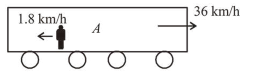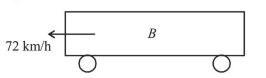# Train A and train B are running on parallel tracks in the opposite directionsQuestion:

Train $A$ and train $B$ are running on parallel tracks in the opposite directions with speeds of $36 \mathrm{~km} /$ hour and $72 \mathrm{~km} /$ hour, respectively. A person is walking in $\operatorname{train} A$ in the direction opposite to its motion with a speed of $1.8 \mathrm{~km} /$ hour. Speed (in $\mathrm{ms}^{-1}$ ) of this person as observed from $\operatorname{train} B$ will be close to : (take the distance between the tracks as negligible)

1. (1) $29.5 \mathrm{~ms}^{-1}$

2. (2) $28.5 \mathrm{~ms}^{-1}$

3. (3) $31.5 \mathrm{~ms}^{-1}$

4. (4) $30.5 \mathrm{~ms}^{-1}$

Correct Option: 1

Solution:

(1) According to question, train $A$ and $B$ are running on parallel tracks in the opposite direction.$V_{A}=36 \mathrm{~km} / \mathrm{h}=10 \mathrm{~m} / \mathrm{s}$$V_{B}=-72 \mathrm{~km} / \mathrm{h}=-20 \mathrm{~m} / \mathrm{s}$

$V_{M A}=-1.8 \mathrm{~km} / \mathrm{h}=-0.5 \mathrm{~m} / \mathrm{s}$

$V_{\operatorname{man}, B}=V_{\operatorname{man}, A}+V_{A, B}$

$=V_{\operatorname{man}} A+V_{A}-V_{B}=-0.5+10-(-20)$

$=-0.5+30=29.5 \mathrm{~m} / \mathrm{s}$